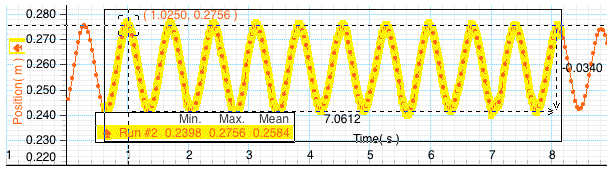# Problem: According to the plot above, what is the equilibrium position as measured by the motion sensor?xo = _____ [m]Answer in meters to the nearest mm OR with N/A if no reasonable answer.

###### FREE Expert Solution

The equilibrium position is the midpoint between the two extreme ends:

$\overline{){{\mathbf{x}}}_{{\mathbf{0}}}{\mathbf{=}}\frac{{\mathbf{x}}_{\mathbf{m}\mathbf{a}\mathbf{x}}\mathbf{+}{\mathbf{x}}_{\mathbf{m}\mathbf{i}\mathbf{n}}}{\mathbf{2}}}$

94% (54 ratings)###### Problem Details

According to the plot above, what is the equilibrium position as measured by the motion sensor?
xo = _____ [m]
Answer in meters to the nearest mm OR with N/A if no reasonable answer.